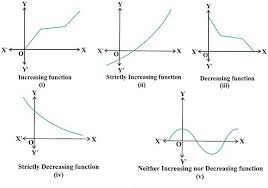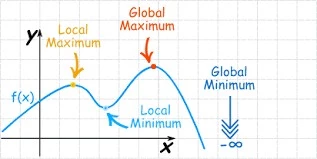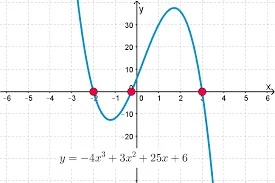or# Application Of Derivatives NCERT Solutions : Class 12 Maths

## NCERT Solutions for Class 12 Maths : Application Of DerivativesFilter
Filters :
Classes
•
•
•
•
•
•
•
Chapters
•
•
•
•
•
•
•
•
•
•
• 3 More
Exercises
•
•
•
•
•
•
•

Doubtnut one of the best online education platform provides free NCERT Solutions of Maths for Class 12 Application Of Derivatives which are solved by our maths experts as per the NCERT (CBSE) guidelines. We provide the solutions as video solutions in which concepts are also explained along with answer which will help students while learning , preparing for exams and in doing homework. These Solutions will help to revise complete syllabus and score more marks in examinations.

Get here free, accurate and comprehensive NCERT Solutions for Class 12 Maths Application Of Derivatives which have been reviewed by our maths counsellors as per the latest edition following up the CBSE guidelines. We provide video solutions in which solutions to all the questions of NCERT Class 12 Maths textbook are explained in step by step and detailed way.

## The Topics of the Chapter Application Of Derivatives are :

APPROXIMATIONS

EQUATION OF TANGENTS AND NORMALS

PROPERTIES OF MAXIMA AND MINIMA

MAXIMA AND MINIMA

TESTS FOR LOCAL MAXIMUM/MINIMUM

APPLICATION OF EXTREMUM

INTERPRETATION OF DY/DX AS A RATE MEASURE

DERIVATIVE AS A RATE MEASURE

TESTS FOR GLOBAL MAXIMUM/MINIMUM

MONOTONOCITY

POINT OF INFLECTION

ANGLE BETWEEN THE CURVES

It contains these exercises along with solved examples. All the exercises are solved in the video. Select the exercise to view the solutions exercisewise of the Chapter Application Of Derivatives:

## NCERT Solutions Class 12 Maths Chapter Application Of Derivatives Exercises:

We have covered all the exercises and also Solved examples in the videos. Along with the practise exercise Students should also practise solved examples to clear the concepts of the Application Of Derivatives. If incase you have any doubt you can watch the solutions for the given questions.Watch the solutions for the given questions in which it is also explained in the video steps to solve the questions along with answers.

# NCERT CLASS 12 SOLUTIONS CHAPTER 6 APPLICATION OF DERIVATIVES

In this chapter, you will come across various applications of derivatives. As in chapter 5 of NCERT book, we dealt with Continuity and Differentiability, until now students must be very much familiar about limits and their uses in a different condition, exponential, and logarithmic function. Therefore, studying the topic Application of derivatives would be easier if the concepts of the previous chapter are clear. Now, we are going to discuss each and everything regarding this chapter in the form of topics and subtopics, making sure the things are explained systematically.

For the authentic content, students should go for NCERT textbook initially, as it has everything a student needs to prepare for their exams. And especially for CBSE students, NCERT is an all-rounder. Almost every examination keeps the NCERT textbook as the reference and the questions in the examination are more or less have their roots from NCERT so one should read and stick to this textbook to do wonders in academics and even in the competition level, by staying well connected to the text throughout the preparation. NCERT maths book has been assembled by the experienced teachers, and everything which is added in the text is important, as it contains the appropriate amount of matter for the students.

Doubtnut provides solution of each unsolved exercise question mentioned in NCERT book. The solutions are provided in the form of videos and pdfs.  It is to bring in the best and the easiest way to learn a chapter effectively.  Doubtnut presents concepts with different graphs and diagrams so that it becomes easier for students to understand through visualization because mugging up thing don’t work in this subject. Students can also visit our website and check our video lectures for deep analysis of the subject as the best teachers are hired for the lectures so that students can score well in examinations and every topic is covered as per the latest CBSE syllabus.

## Topics and Subtopics of NCERT Maths Class 12 Chapter 6 – Application of Derivatives

Let us know the topics and subtopics of NCERT Maths Class 12 Chapter 6, Application of Derivatives:

### Rate of change of quantities

It is the rate of change of quantities with respect to other quantities.

#### MONOTONICITYA function is said to be monotonic if it’s either increasing or decreasing in its domain.

Strictly increasing -(y=f(x))

In the graph, we can see a strictly increasing function

Such a function is called a strictly increasing function or monotonically increasing function.

That is it is constantly increasing in its domain.

Strictly decreasing function -In the graph, we can see a strictly decreasing function.  Such a function is called a strictly decreasing function or monotonically decreasing                  function as it is constantly decreasing in its domain.

Function and their behaviour

Function                                                        Behaviour

f(x) = x                                                          strictly increasing on R

f(x) = x²                                                         strictly decreasing on(–∞ , 0]

strictly increasing on [0,∞)

f(x) = √x                                                        strictly increasing on [0, ∞)

f(x) = x³                                                         strictly increasing on R

f(x) = IxI                                                        strictly decreasing on (-∞,o]

strictly increasing on [ 0, ∞)

f(x) = 1/x                                                      neither deceasing nor increasing on R .

strictly decreasing on (-∞, 0) , strictly decreasing

on(0, ∞)

f(x)= [x]                                                         increasing on R

f(x)= {x}                                                         neither increasing nor decreasing on R.

However, strictly increasing on [n, n+1) where n Ïµ Z

f(x) = sin x                                                    neither increasing nor decreasing on R

strictly increasing on [ (2n – 1/2)] π], (2n +1/2)π]; nÏµZ

strictly decreasing on [(2n+ 1/2)π, (2n + 3/2)π] ; nÏµZ

CRITICAL POINTS

It is a collection of points for which,

1. f(x) does not exist

2. f'(x) does not exist

3. f’(x) = 0

All the values of x obtained from the above condition are said to be critical points.

Note: Critical points are the interior points of an interval.

COMPARISON OF FUNCTION USING CALCULUS:

If for instance, we want to compare á¶²(x) and g(x) then we consider a function f(x)=á¶²(x)-g(x) or f(x) or f(x) = g(x) - á¶²(x)  and check whether f(x) is increasing or decreasing in given domain of á¶²(x) and g(x) .

#### MAXIMA AND MINIMA

Introduction to maxima and minima -

By the maximum/minimum value of function f(x), we mean local or regional maximum/minimum value and not the greatest / least value attainable by the function. It is also possible in a function that local maximum at one point is smaller than the local minimum at another point.

The words used here is called extrema for maxima and minima.

Definition:A function f(x) is said to have a maximum at x=a if f(a) is greatest of all values in the suitably small neighborhood of a, where x= a is an interior point in the domain of f(x).

### METHODS OF FINDING EXTREMA OF CONTINUOUS FUNCTION

The following tests apply to a continuous function in order to get the extrema-

#### FIRST DERIVATIVE TEST FOR FIRST MAXIMA AND MINIMA

The function attains maximum when it has assumed its maximum value and attains minimum when it has assumed its minimum value which could be shown as -

THEOREM 1.  First derivative test -

Let f be a differentiable function defined on an interval I and let a Ïµ I.

Then,

1. x=a is a point of the local maximum value of f if

i. f’(a) =0

ii. f'(x) changes the sign from positive to negative as x increases through “a” that is f'(x)>0 at every point sufficiently close to and to the left of “a”, and f'(x) < 0 at every point sufficiently close to the right of “a”.

2. x= a is a point of local minimum value of f, if

i. f’(a) = 0

ii. f'(x) changes the sign from negative to positive as x increases through a that is f'(x)<0 at every point sufficiently close to and to the left to a, and f'(x) >0 ay every point sufficiently close to and to the right of a.

3. If f’(a) = 0 and f'(x) does not change sign as increases through a that is, f'(x) has the same sign in the complete neighbourhood of a, then a is neither a point of local maximum value nor a point of local minimum value. In fact, such a point is called INFLEXION.

nth Derivatives Test:

First we find the root of f’(x) = 0. Suppose x = a is one of the roofs of f’(x) = 0

Now, find f’’(x) at x = a

i. if f’’ (a) = negative, then f(x) is maximum at x= a

ii. if f’’ (a) = positive, then f(x) is minimum at x= a

iii. If f’’ (a) zero

Then we find f’’’ (x) at x= a

If f’’’ (a) 0, then f(x) has neither maximum nor minimum (inflexion point), at x= a.

But if f’’’ (a) = 0, then find f(iv)(a)

if f(iv)(a) = positive , then f(x) is minimum at x = a

if f(iv) (a) = negative , then f(x) is maximum at x= a , and so on , process is repeated till point is discussed.

#### MAXIMA AND MINIMA OF DISCONTINUOUS FUNCTION

In discontinuous functions, we don’t apply any of the methods discussed earlier, but we observe certain conditions:

#### MINIMUM OF DISCONTINUOUS FUNCTIONS

Case 1- f(a)

Case 2- f(a)

Case 3- f(a)f(a-h)

Case 4- f(a)f(a+h)           f(a)

From all the four cases discussed earlier for minimum of discontinuous functions, we have

f(a) f(a+h) and f(a)f(a-h)

#### MAXIMUM OF DISCONTINUOUS FUNCTIONS

Let f(x) be a function discontinuous or not differentiable at x=a, then the following four cases arises from maximum at x= a

1. f(a)>f(a+h)      f(a)>f(a-h)

2. f(a)>f(a+h)      f(a)>f(a-h)

3. f(a)>f(a+h)      f(a)f(a-h)

4. f(a)f(a+h)      f(a)>f(a-h)

5. f(a)f(a+h)

6. f(a)f(a+h)

### NATURE OF ROOTS OF CUBIC POLYNOMIAL

Let f(x) = ax³ + bx²+cx + d be the given cubic polynomial and f(x) =0 be the corresponding cubic equations, where a, b, c, d Ïµ R and a>0

Now, f’(x) = 3ax²+2bx +c

Let D= 4b²-12ac = 4(b²-3ax) be the discriminant of the equations f’(x) = 0

Case-1

If D<0 f’(x) >0, for all xÏµ R, that is f(x) would be an increasing function of x. Also, lim(x) f(x) = and lim(x-) = -

Since, f(x) is an increasing function, so it will intersect the x- axis at only one place.

Case-2

If D > 0 f’(x) = 0 would have two real roots (say)

É‘ and β (where É‘<β)

f’(x) = 3a(x-É‘)(x-β)

f’(x) < 0, xÏµ(É‘,β)

f’(x)> 0 , xÏµ(-∞, É‘) U (β,)

f’(x)= 0 , x Ïµ{É‘,β}

Here, x=É‘ , will be point of local maxima and x=β will be point of local minima.

Case-3

If D=0 , f’(x) = 3a (x-É‘)², where É‘ is a root of

f’(x) = 0 , then f(x) = a (x-É‘)³+ K  . If K = 0 , then

f(x)= 0 has three equal real roots . If K 0 , Then

f(x) = 0 has one real root .

### GRAPHICAL REPRESENTATION OF ROOTS OF CUBICAL POLYNOMIAL## Significance of NCERT Mathematics Book Class 12

1. NCERT book for Class 12 is organized and represented in a systematic manner for the convenience of the students.
2. NCERT book also provides illustrations almost after every topic for the quick revision for the students.
3. For the interest of the students and motivational purposes, a brief about the scientists is provided in the beginning and at the end of the chapter to know the basic, origin and developer of the particular topic.

For more, students should visit our website to discover a fun way to learn the subject with ease. As Doubtnut provides the solution for all the chapters in a video as well as in a written format which makes it quite easier for students to grasp the concepts. Moreover, students can always clear their doubts by posting queries on the same, which are replied by senior professionals and experts of mathematics.